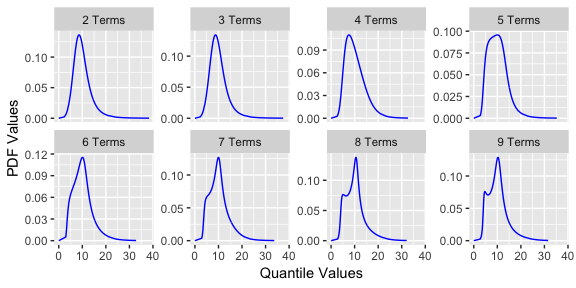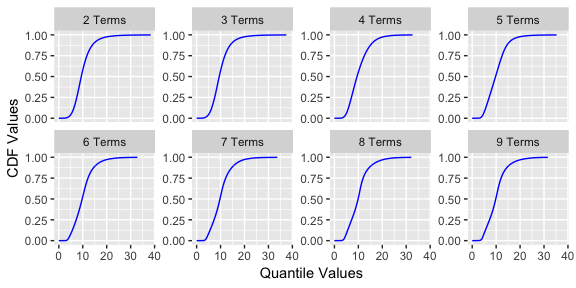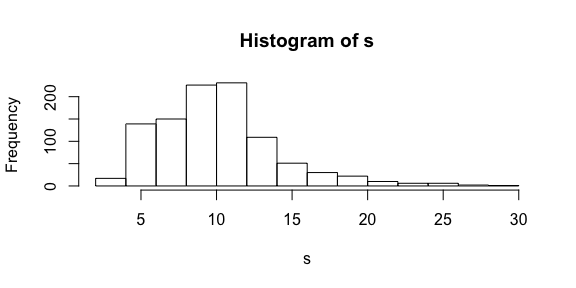# Metalog Distributions in R

### The R Metalog Distribution

This package generates functions for the metalog distribution. The metalog distribution is a highly flexible probability distribution that can be used to model data without traditional parameters.

### Metalog Background

In economics, business, engineering, science and other fields, continuous uncertainties frequently arise that are not easily- or well-characterized by previously-named continuous probability distributions. Frequently, there is data available from measurements, assessments, derivations, simulations or other sources that characterize the range of an uncertainty. But the underlying process that generated this data is either unknown or fails to lend itself to convenient derivation of equations that appropriately characterize the probability density (PDF), cumulative (CDF) or quantile distribution functions.

The metalog distributions are a family of continuous univariate probability distributions that directly address this need. They can be used in most any situation in which CDF data is known and a flexible, simple, and easy-to-use continuous probability distribution is needed to represent that data. Consider their uses and benefits. Also consider their applications over a wide range of fields and data sources.

This repository is a complement and extension of the information found in the paper published in Decision Analysis and the website

### Using the package

Once the package is loaded, start by fitting a dataset of observations from a continuous distribution. For this vignette, we will load the library and use an included example of fish (steelhead trout) weight measurements from the Pacific Northwest. This data set is illustrative to demonstrate the flexibility of the metalog distribution as it is bi-modal. The data is installed with the package. Steelhead trout, unlike salmon, return to fresh water multiple times in their lives. However, with a traditional distribution, it is difficult to see the difference in size of between the one salt or two salt (salt being the number of times a fish returned to the ocean).

library(rmetalog)

data("fishSize")
summary(fishSize)
##     FishSize
##  Min.   : 3.00
##  1st Qu.: 7.00
##  Median :10.00
##  Mean   :10.18
##  3rd Qu.:12.00
##  Max.   :33.00

The base function for the package to create distributions is:

metalog()

This function takes several inputs:

• x - vector of numeric data
• term_limit - integer between 3 and 30, specifying the number of metalog distributions, with respective terms, terms to build (default: 13)
• bounds - numeric vector specifying lower or upper bounds, none required if the distribution is unbounded
• boundedness - character string specifying unbounded, semi-bounded upper, semi-bounded lower or bounded; accepts values u, su, sl and b (default: ‘u’)
• term_lower_bound - (Optional) the smallest term to generate, used to minimize computation must be less than term_limit (default is 2)
• step_len - (Optional) size of steps to summarize the distribution (between 0.001 and 0.01, which is between approx 1000 and 100 summarized points). This is only used if the data vector length is greater than 100.
• probs - (Optional) probability quantiles, same length as x

Here is an example of a lower bounded distribution build.

my_metalog <- metalog(fishSize$FishSize, term_limit = 9, bounds=0, boundedness = 'sl', step_len = .01) The function returns an object of class rmetalog and list. You can get a summary of the distributions using summary. summary(my_metalog) ## ----------------------------------------------- ## Summary of Metalog Distribution Object ## ----------------------------------------------- ## ## Parameters ## Term Limit: 9 ## Term Lower Bound: 2 ## Boundedness: sl ## Bounds (only used based on boundedness): 0 33 ## Step Length for Distribution Summary: 0.01 ## Method Use for Fitting: any ## ## ## Validation and Fit Method ## term valid method ## 2 yes OLS ## 3 yes OLS ## 4 yes OLS ## 5 yes OLS ## 6 yes OLS ## 7 yes OLS ## 8 yes OLS ## 9 yes OLS The summary shows if there is a valid distribution for a given term and what method was used to fit the data. There are currently only two methods traditional least squares (OLS) and a more robust constrained linear program. The package attempts to use OLS and if it returns an invalid distribution (described in the literature referenced above) tries the linear program. You can also plot a quick visual comparison of the distributions by term. plot(my_metalog) ##$pdf##
## \$cdfAs the pdf plot shows with a higher number of terms, the bi-modal nature of the distribution is revealed which can then be leveraged for further analysis. Once the distributions are built, you can create n samples by selecting a term.

s <- rmetalog(my_metalog, n = 1000, term = 9)
hist(s)You can also retrieve quantile, density, and probability values similar to other R distributions.

qmetalog(my_metalog, y = c(0.25, 0.5, 0.75), term = 9)
##   7.236934  9.840370 12.062076

probabilities from a quantile.

pmetalog(my_metalog, q = c(3,10,25), term = 9)
##  0.001953843 0.520067119 0.992283776

density from a quantile.

dmetalog(my_metalog, q = c(3,10,25), term = 9)
##  0.004544097 0.126974552 0.002289697

Any feedback is appreciated! Please submit a pull request or issue to the development repo if you find anything that needs to be addressed.